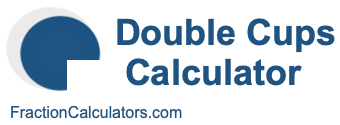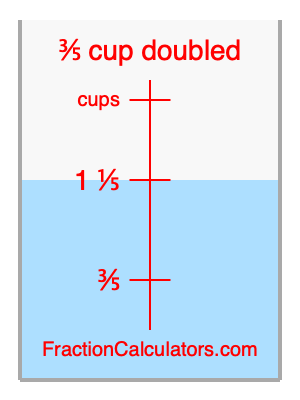What is 3/5 cup doubled?Here we will calculate 3/5 cup doubled and illustrate 3/5 cup doubled on a measuring cup. What is 3/5 cup doubled? 3/5 cup doubled is twice 3/5 cup. Therefore, to double 3/5 cup, we multiply 3/5 by 2. Here is the formula, the math, and the answer to 3/5 cup doubled:

Cup × 2 = Cup doubled
3/5 × 2 = 1 1/5
3/5 cup doubled = 1 1/5 cups

Below is an illustration of a measuring cup. We show 3/5 cup and 3/5 cup doubled (1 1/5 cups) so you can see where they are in relation to each other.As you can see, we filled up our measuring cup to 3/5 cup doubled. In other words, we filled it up to 1 1/5, which is two times 3/5.

Double Cups Calculator
Here you can double another cup measurement.

What is cups doubled?

What is 5/8 doubled?
Here is the next measurement on our list that we have doubled for you.